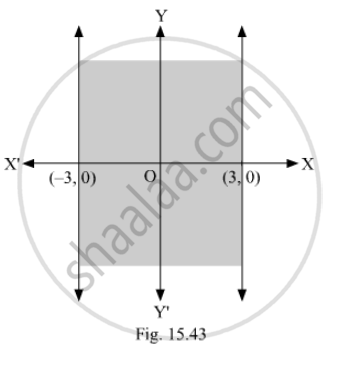Advertisement Remove all ads

# Mark the Correct Alternative in Each of the Following: the Inequality Representing the Following Graph is - Mathematics

MCQ

Mark the correct alternative in each of the following:
The inequality representing the following graph is

#### Options

• $\left| x \right|$$<$3

• $\left| x \right|$$\leq$3

• $\left| x \right|$$>$3

• $\left| x \right|$$\geq$Advertisement Remove all ads

#### Solution

As according to the graph,

$\text{ x lies between } - 3 \text{ and } 3$
$\Rightarrow - 3 \leq x \leq 3$
$\Rightarrow \left| x \right| \leq 3$

Hence, the correct option is (b).

Is there an error in this question or solution?
Advertisement Remove all ads

#### APPEARS IN

RD Sharma Class 11 Mathematics Textbook
Chapter 15 Linear Inequations
Q 8 | Page 32
Advertisement Remove all ads
Advertisement Remove all ads
Share
Notifications

View all notifications

Forgot password?